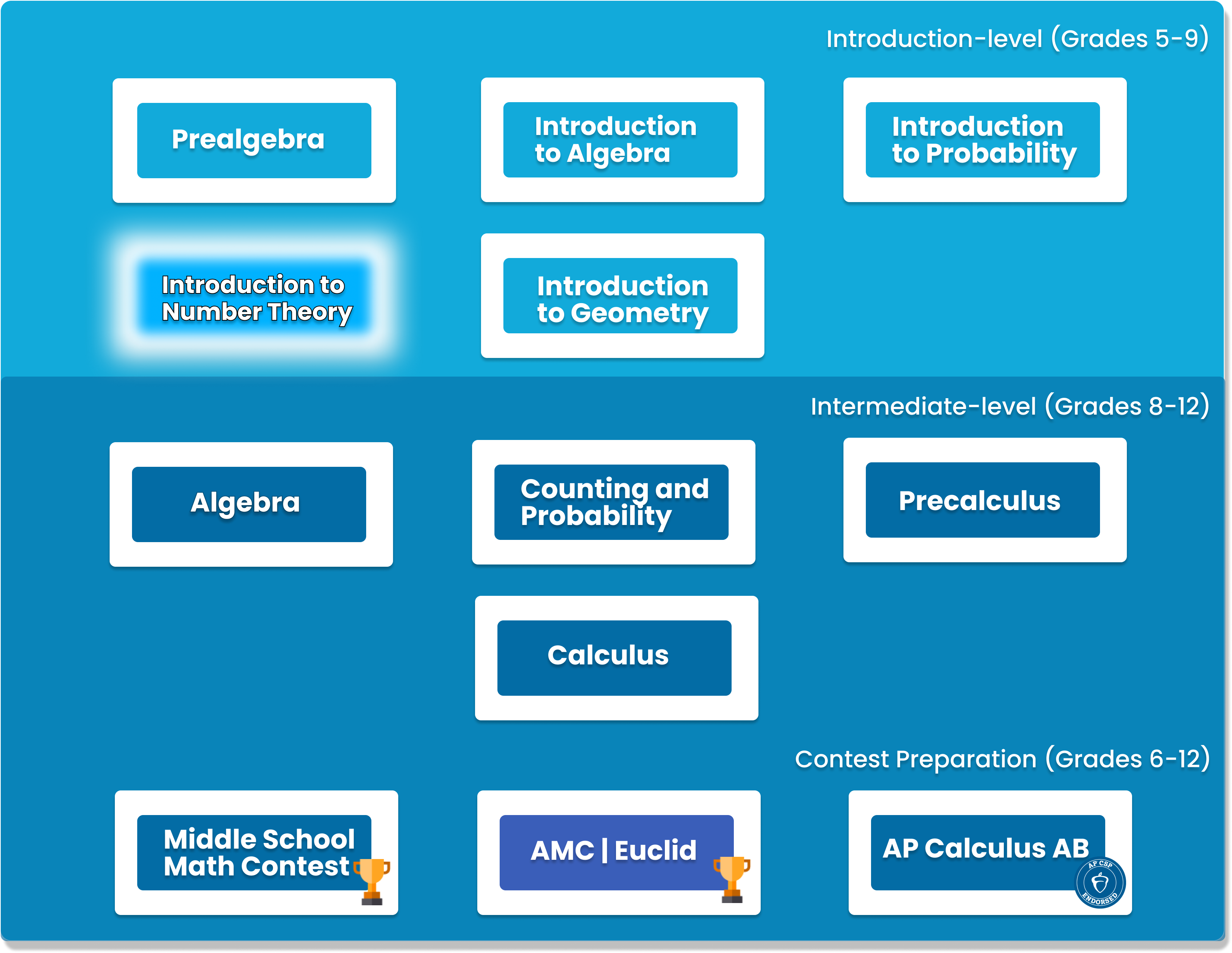# Introduction to Number Theory

This course is designed to build the basics of a complicated mathematical field. It is designed to be a complete introduction to the fundamental concepts of number theory for students in grades 7-10. The class covers topics such as primes and composites; multiples, divisors, and divisibility; prime factorization and its uses; modular arithmetic and more.##### Who is this course for
This Course is for Grade 5-Grade 9 Students who want to advance their knowledge in Number Theory. And also for students who want to challenge themselves in middle school competitions.
This course typically takes 3-6 months to complete. This depends on the student's experience and how fast they can master the concepts and the knowledge. Our instructors move at the pace of the student, it may take extra time for some students to reinforce what they have learned.
##### Curriculum
###### Chapter 1: Integers: The BasicsMultiples and Divisors
###### Chapter 2: Primes and CompositesPrimes, Composites, and identifying prime numbers
###### Chapter 3: Multiples and DivisorsGreatest Common Divisors and Least Common Multiples, Euclidean algorithm
###### Chapter 4: Prime FactorizationFactorization of numbers, application problems
###### Chapter 5: Divisor ProblemsCounting the number of divisors of a number
###### Chapter 6: Special NumbersFactorials, Divisibility, Palindromes
###### Chapter 7: Algebra with IntegersSolving algebraic equations that only involve integers
###### Chapter 8: Base NumberNumber in bases other than base 10, conversion of different bases
###### Chapter 9: Base Number ArithmeticAddition, subtraction, multiplication and division of number in different bases
###### Chapter 10: Unit DigitsSolving problems with unit digits of numbers
###### Chapter 11: Decimals and FractionsTerminating and repeating decimals, conversion of decimals to fractions
###### Chapter 12: Introduction to modular arithmeticCongruence, addition and multiplication of remainders
###### Chapter 13: Divisibility RulesLearn to quickly check if a number is divisible by 2, 3, 4, 5, 7, 9, 11, 13
###### Chapter 14: Linear CongruencesSolving linear congruence equations
###### Chapter 15: Number SenseFamiliarize with numbers to solve problems efficiently

### You Might Also Be Interested In Our Elective Courses##### Python Level 1
AGES 10 - 15

Designed for beginners, this level is designed to teach the basic fundamentals and design principles of Python, with the help of Turtle graphics and PyGame. This includes: variables, conditional statements, loop basics, and functions.

These concepts are transferable to any other programming language. Throughout the way, students will create projects in order to apply the concepts they have learned, and to solidify their knowledge.##### Python Level 2
AGES 10 - 15

Level 2 dives deeper into the basics of Python for a more thorough understanding and introduces advanced topics. Students will build on knowledge from Level 1 and work with data structures, advanced loops, algorithms, and object-oriented programming, and create games based on what they learn.

Students will complete the course with a solid understanding of Python fundamentals.##### Java Level 1
AGES 10 - 15

This beginner-friendly course serves as an introduction to the Java programming language. Students will learn the fundamentals of Java along with core computer science concepts. Concepts covered in this course include Java syntax, types and identifiers, operators, if statements, loops, arrays and more.

Students will apply these concepts to create fun games such as Tic-Tac-Toe.